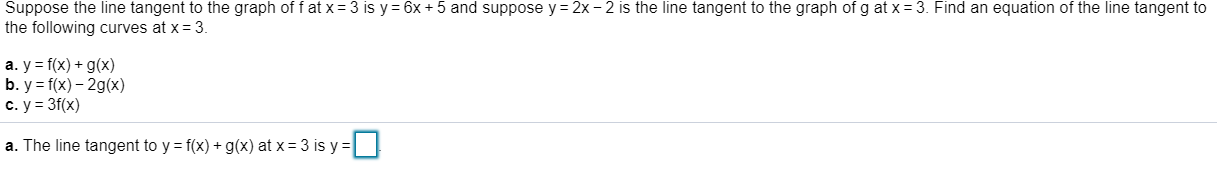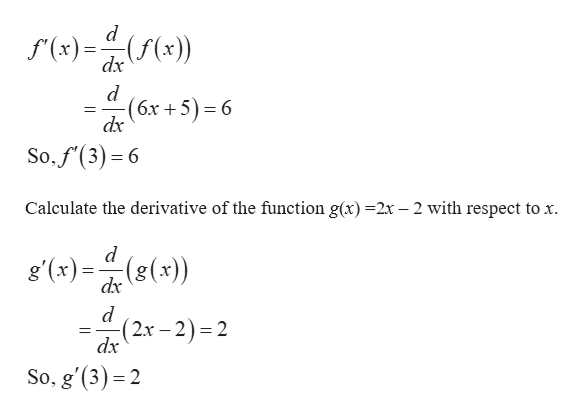Suppose the line tangent to the graph of f at x3 is y 6x 5 and suppose y2x - 2 is the line tangent to the graph of g at x3. Find an equation of the line tangent tothe following curves at x- 3.a. y f(x) g(x)b. y f(x)-2gx)c. y 3f(x)a. The line tangent to y = f(x) + g(x) at x = 3 is y = |

Questionhelp_outlineImage TranscriptioncloseSuppose the line tangent to the graph of f at x 3 is y 6x 5 and suppose y 2x - 2 is the line tangent to the graph of g at x 3. Find an equation of the line tangent to the following curves at x- 3. a. y f(x) g(x) b. y f(x)-2gx) c. y 3f(x) a. The line tangent to y = f(x) + g(x) at x = 3 is y = | fullscreen
Step 1

The tangent line to the graph of f at the point x=3 is y = 6x + 5 and the tangent line the graph of g at the point x=3 is y = 2x – 2.

Calculate ...help_outlineImage Transcriptionclosed (x) x)) dx -(6х +5) - 6 dx So.f(3)6 Calculate the derivative of the function g(x)=2x - 2 with respect to x. d g' (x)((x)) dx (2x-2)2 dx So, g'(3 2 - fullscreen

Want to see the full answer?

See Solution

Want to see this answer and more?

Our solutions are written by experts, many with advanced degrees, and available 24/7

See Solution
Tagged in

Other